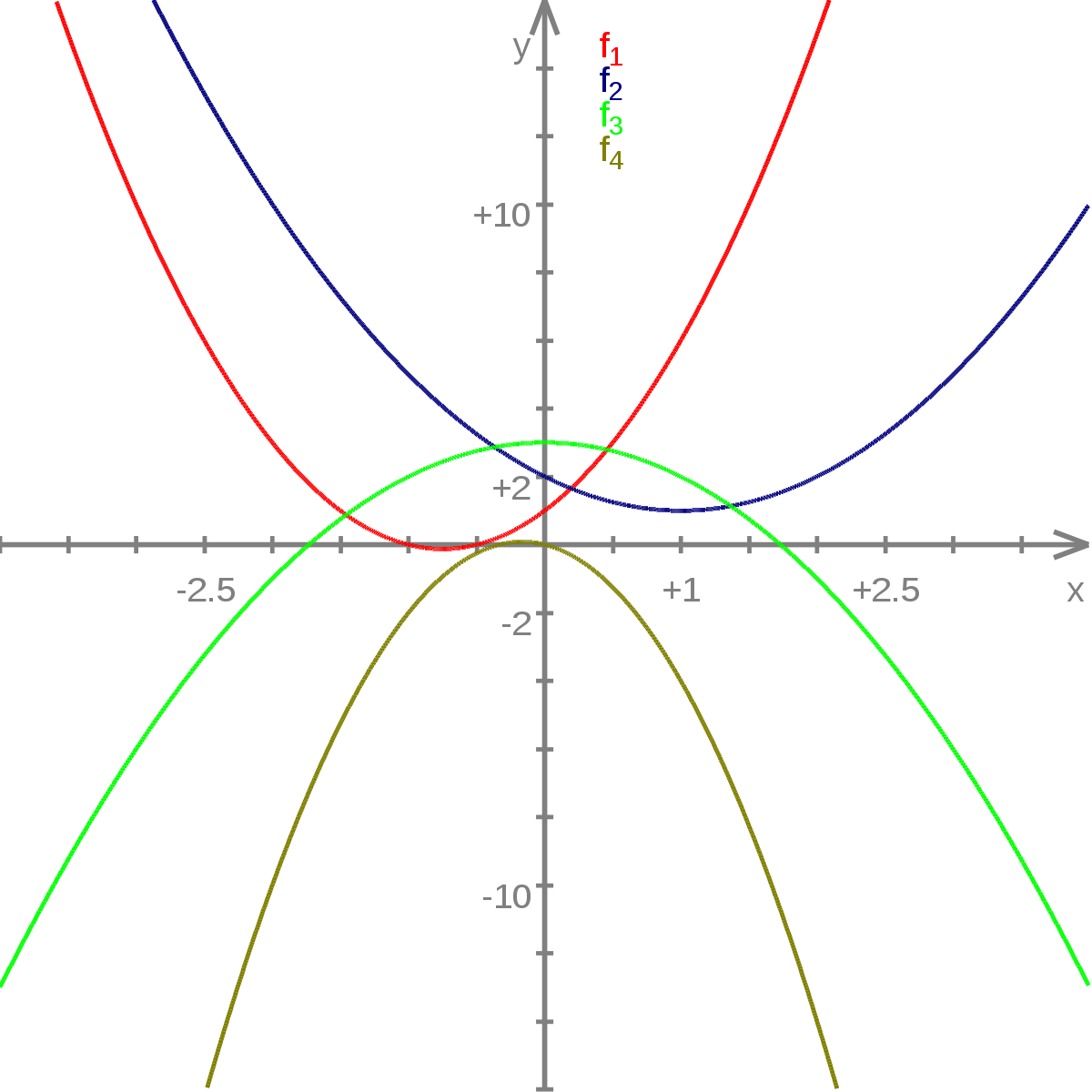# Second Derivative Calculator

Enter the function and choose the variable. Hit the Calculate button to find the second derivative using second derivative calculator.

This will be calculated:

Give Us Feedback

## Second Derivative Calculator

A second derivative calculator is an online tool that performs differentiation twice on a function. It can find both first and second derivatives.

Moreover, the 2nd derivative calculator gives the complete solving process with a step-by-step solution.

## How does the second derivative calculator work?

The second derivative test calculator is an easy-to-use tool. Follow these steps to find the second derivative.

1. Enter the function.
2. Choose the variable.
3. Confirm the displayed function from the display box.
4. Click calculate.

To understand the differentiation procedure, click on the ‘+’ icon in the results. It will give a step-wise guide. You can also download the ‘PDf’ copy of the detailed result.

## What is the second derivative?

The derivative taken of the same function for the second time is known as the second derivative. It is the same as the first derivative except for the notation.

The second derivative is represented by two dots over the variable or two dashes on f in the notation f(x) e.g f’’(x).

A graphical representation of 2nd derivatives can be seen below.## How to find the second derivative?

There is no separate process or formula for the second derivative. It is the same as the first. The second derivative is differentiation performed on the derivative of a function.

Let’s see an example of the second derivative.

Example 1:

Calculate the second derivative for function x = Sinx + x2

Solution:

Step 1: Arrange the function.

f(x) = x2 + sinx

Step 2: Find the first derivative.

f’(x) = d/dx [x2 + sinx]

f’(x) = d/dx [x2] + d/dx[sinx]

f’(x) = 2x + cosx

Step 3: Find the 2nd derivative.

f’’(x) = d/dx [2x + cosx]

f’’(x) = d/dx [2x] + d/dx[cosx]

f’’(x) = 2 - sinx

Example 2:

Find the second derivative for a*(x2+b).

Solution:

First derivative.

Step 1: Apply derivative.

f’(x)= d/dx [a*(x2+b)]

Step 2: Take constant out.

f’(x)= a d/dx (x2 + b)

Step 3: Apply constant rule and power rule.

f’(x)= a (2*x2-1 + 0)

f’(x)= a (2x + 0)

f’(x)= 2ax

Second Derivative:

Step 4: Apply the second derivative.

f’’(x) = d/dx (2ax)

Step 5: Take the constant out.

f’’(x) = 2a d/dx (x)

f’’(x) = 2a (x1-1)

f’’(x) = 2a

Example 3:

What is the second derivative of sinx + x/2?

Solution:

First derivative:

Step 1: Apply the derivative.

f’(x) = d/dx (sinx + x/2)

Step 2: Apply the Sum rule.

f’(x) = d/dx(sinx) + d/dx(x/2)

Step 3: Take out the constant.

f’(x) = cosx + (½)d/dx(x)

f’(x) = cosx + ½

Second derivative:

Step 4: Apply the second derivative.

f’’(x) = d/dx (cosx + ½ )

Step 5: Apply the sum rule.

f’’(x) = d/dx (cosx) + d/dx(½)

Step 6: Constant rule.

f’’(x) = -sinx + 0

### 📋 Related Blogs